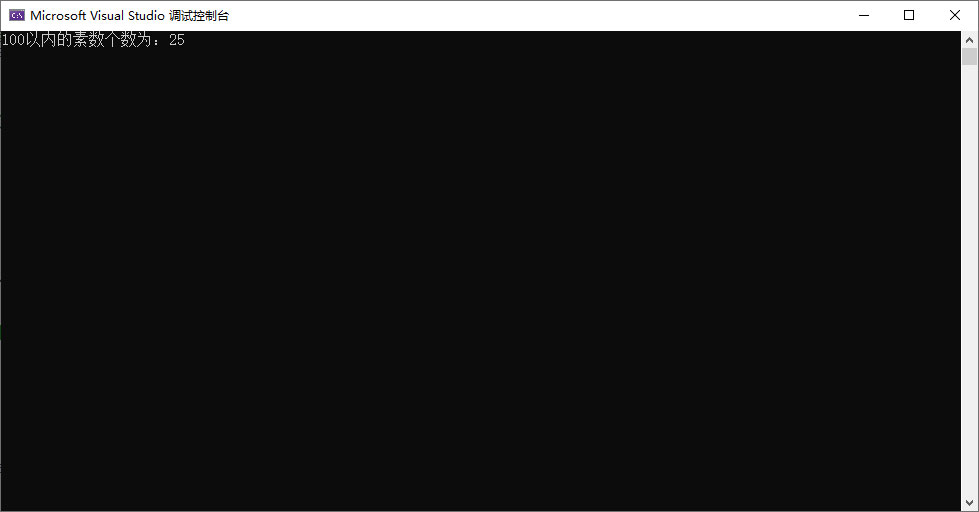﻿ C#实现统计100以内所有素数的个数_C#教程_脚本之家
C#教程# C#实现统计100以内所有素数的个数

## 代码实现

```//最大范围内数字
int MaxNum = 100;
//所有素数和，初始0
int sum = 0;

//2是最小的素数，for循环范围为2-100
for (int i = 2; i <= MaxNum; i++)
{
if (if_prime(i))
{
//统计所有素数之和
sum++;
}
}

//判断数字是否为素数的方法
bool if_prime(int num)
{
int i = 0;
for (i = 2; i < num; i++)
{
/* 如果该数有存在1以外的其他正因数，则不是素数 */
if (num % i == 0)
return false;
}
return true;
}
Console.WriteLine(MaxNum + "以内的素数个数为：" + sum);```

## 运行结果